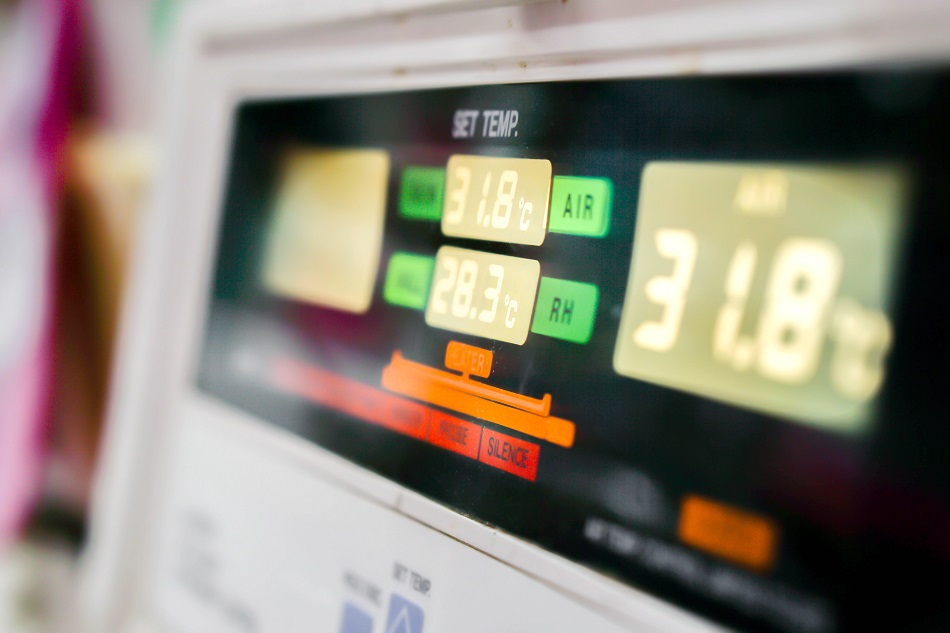Editorial Feature

# An Introduction to Silicon Bandgap Temperature SensorsImage Credit: Atiwat Witthayanurut/Shutterstock.com

A silicon bandgap temperature sensor is a type of thermometer or temperature detector commonly employed in electronic devices. They have good stability at extreme environmental conditions due to the integral stability of crystalline silicon.

Silicon bandgap temperature sensors are generally manufactured using photolithographic methods.

## Working Principle of Silicon Bandgap Temperature Sensors

Silicon bandgap temperature sensors are operated on a principle of temperature dependence of forward voltage of a silicon diode, which is represented by the following equation:

VBE = VG0(1 - T / T0) + VBE0(T / T0) + (nKT / q) ln(T0 / T) + (KT / q)ln(IC / IC0)

where:

n = a device-dependent constant
q = charge on an electron
K = Boltzmann's constant
VBE0 = bandgap voltage at temperature T0 and current IC0
VG0 = bandgap voltage at absolute zero
T = temperature in K

Most of the variables in the above equation can be eliminated by comparing the bandgap voltages at two different currents, IC1 and IC2. This results in the following equation:

ΔVBE = (KT / q)ln(IC1 / IC2)

Thus, ΔVBE can be measured with an electronic circuit that is used to calculate the temperature of the diode. The output remains stable at temperatures of approximately 200 to 250°C. More exotic materials like silicon carbide can be employed for temperatures above 250°C.

## Advantages of Silicon Bandgap Temperature Sensors

The key benefits of silicon bandgap temperature sensors are:

• Low cost
• Accurate and reliable
• Highly consistent measurements
• Positive temperature coefficient

## Applications of Silicon Bandgap Temperature Sensors

The major applications of silicon bandgap temperature sensors include:

• Overheating protection
• White goods and power supplies
• Heating systems and air-conditioning
• Engine oil and coolant
• Transmissions